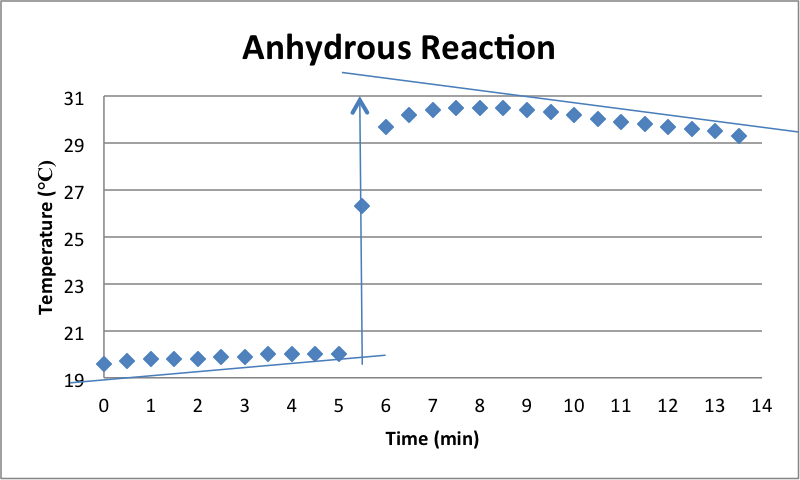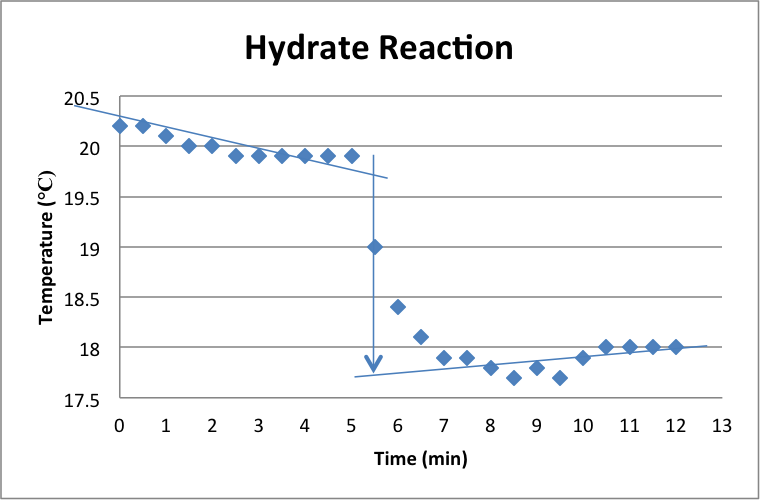# Enthalpy of Hydration

Written by Rachel Buckley

Abstract

This experiment, Enthalpy of Hydration, is used to calculate the enthalpy of hydration of magnesium sulfate by measuring the temperature change of both anhydrous magnesium sulfate and hydrated magnesium sulfate when they are added separately to two different calorimeters and allowed to react. The results are then used to calculate the enthalpy of hydration.

Methods and Materials

First, one sample of anhydrous, between 7 and 8 grams, and one sample of hydrate, between 15.3 and 15.4 grams, were measured out to four significant figures. Then, a weighed polystyrene cup was obtained and filled with 100 mL of distilled water. The thermometer attached to the Palm Pilot was then placed in the water and allowed to obtain measurements for 5 minutes. After five minutes, the anhydrous was rapidly added to the water, which was constantly being stirred, and allowed to react for an additional 8 minutes, or until the temperature became pretty constant. The Palm Pilot recorded the temperature every thirty seconds during this time period. The same process was repeated with the hydrate, using new distilled water.

Observations and DiscussionsThe graph above shows the change in temperature over a 14-minute time period for the reaction of the anhydrous magnesium sulfate. The jump in the graph represents the time at which the anhydrous was added to the distilled water. The water eventually started cooling back down after it heated up due to the dissolution of the anhydrous that was added to the water. The increase in temperature indicates that the reaction involved is exothermic, thus releasing energy and heating up. The temperature change over the whole reaction was 9.7 °C. This number was then plugged into the equation Q=-CΔT, where Q is the heat change, C is the heat capacity of the system, and ΔT is the observed temperature change. The heat capacity of the system was found by multiplying the mass of the anhydrous plus the mass of the water, 107.634 g, by the specific heat of the mixture, 3.84 J/g°C. Once heat capacity was calculated, it was plugged into the equation above. The calculated heat change of the reaction was -4009.15 J. This number was then divided by the number of moles of solute to find the enthalpy of dissolution, which ended up being -63.21 kJ/mol.The graph above shows the temperature over a 13-minute period for the reaction of the hydrated magnesium sulfate. The dip in the graph was due to the addition of the hydrate after five minutes of the water adjusting. The decrease in temperature represents the fact that the hydrate requires energy to dissolve, thus the reaction is an endothermic reaction. The change in temperature for the reaction was -2.2 °C. The heat of dissolution was calculated in the same manner as it was for the anhydrous, where the mass of the hydrate mixture was 115.34 g, the heat capacity was 442.9, and the heat change of the reaction was 974.39 J. This number was divided by 0.062237 mol, the moles of solute added. This yields the enthalpy of dissolution, which was 15.65 kJ/mol. Finally, to find the enthalpy of hydration, the enthalpy of dissolution of the hydrate was subtracted from the enthalpy of dissolution of the anhydrous. The calculated enthalpy of hydration for this experiment was -78.86 kJ/mol.

The enthalpy of hydration can be calculated by subtracting the two enthalpies of dissolution of the anhydrous and the hydrate due to Hess’s Law, which states that if a particular chemical reaction can be expressed as the sum of two or more other chemical reactions, the enthalpy changes, or heat change, will also be the sum of enthalpy changes of the other chemical reactions. The enthalpy of dissolution of the hydrate can be added to the enthalpy of hydration of the overall reaction to find the enthalpy of dissolution of the anhydrous, so that equation can be rearranged such that the enthalpy of dissolution of the hydrate may be subtracted from the enthalpy of dissolution of the anhydrous to find the enthalpy of hydration for the overall reaction. This law is advantageous due to the fact that enthalpies of hydration are difficult to measure directly.

Conclusion

The experiment performed, Enthalpy of Hydration, was used to determine the enthalpy of hydration of an anhydrous magnesium sulfate sample. This was found by measuring the temperature change over a period of time for a reaction of water and the anhydrous sample, as well as the reaction of a hydrated magnesium sulfate sample. The end result yielded the enthalpy of -78.86 kJ/mol. There are no known exact enthalpies of hydration, due to the fact that they are difficult to measure directly.

• Share